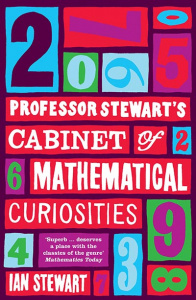## Professor Stewart’s Cabinet of Mathematical CuriositiesBy Ian Stewart
Review by Matthew (Mathematics)

Most people’s first experience of a “maths book” is a thick tattered school textbook filled with tedious exercises. With such a book it is easy to get bored and overlook the beauty of mathematics. However, one just needs to dig a little deeper to realise that very little of maths involves monotonous calculations (after all we have computers for that!). I discovered this with the help of Professor Stewart’s Cabinet of Mathematical Curiosities.

This book isn’t what you would think of when I say book, it is more a collections of problems and curiosities from all areas of mathematics. The entries in this book are rarely more than two pages long so can be quickly looked at whenever you have a bit of spare time; however some of the problems in the book are deceptively simple (indeed some are yet to be solved!) and can leave you thinking about them for days.

One day I flipped to an entry called Pick’s Theorem and saw that if a polygon whose vertices lie on the points of a square lattice (as in the picture), where each point is spaced at interval of one unit, then the area of the polygon can be calculated from the number of boundary points B (red) and interior points (blue) by the formula = 1/2B + I – 1. (In this example B = 15 and = 31 so A = 15/2 + 31 – 1 = 37.5) I felt that this theorem was wonderfully beautiful and simple and I went onto the internet (i.e. Wikipedia!) and read through a proof of it.

As I said before sometimes at school I thought that maths got tedious and repetitive. I struggled however to find any good maths book because many maths books are just textbooks in disguise. This often made me doubt whether maths was really the right choice for me at university; after all what if maths at university was just going to be manipulating large numbers and solving complicated equations? This book made discover the diversity of areas of mathematics and realise that my impression of university mathematics was very from the reality.

There is a rather tantalising entry in the book entitled How to Win a Million Dollars by Proving the Obvious, which discuss the P = NP computer science open problem that turns out to be “not that obvious”. The book also contains an acronym from the science fiction author Robert A. Heinlein: TANSTAAFL – There Ain’t No Such Thing As A Free Lunch!

Be warned though this book also includes some (rather poor) mathematical jokes (I would skip over them!)

Professor Stewart’s Cabinet of Mathematical Curiosities by Ian Stewart
ISBN-10: 1846683459
ISBN-13: 9781846683459

Try checking the availability of this book at your school or local library or explore second hand bookshops and websites. You may also wish to purchase from either Amazon or Blackwell’s.

## Contact Univ

If you have any questions or need more information, just ask: